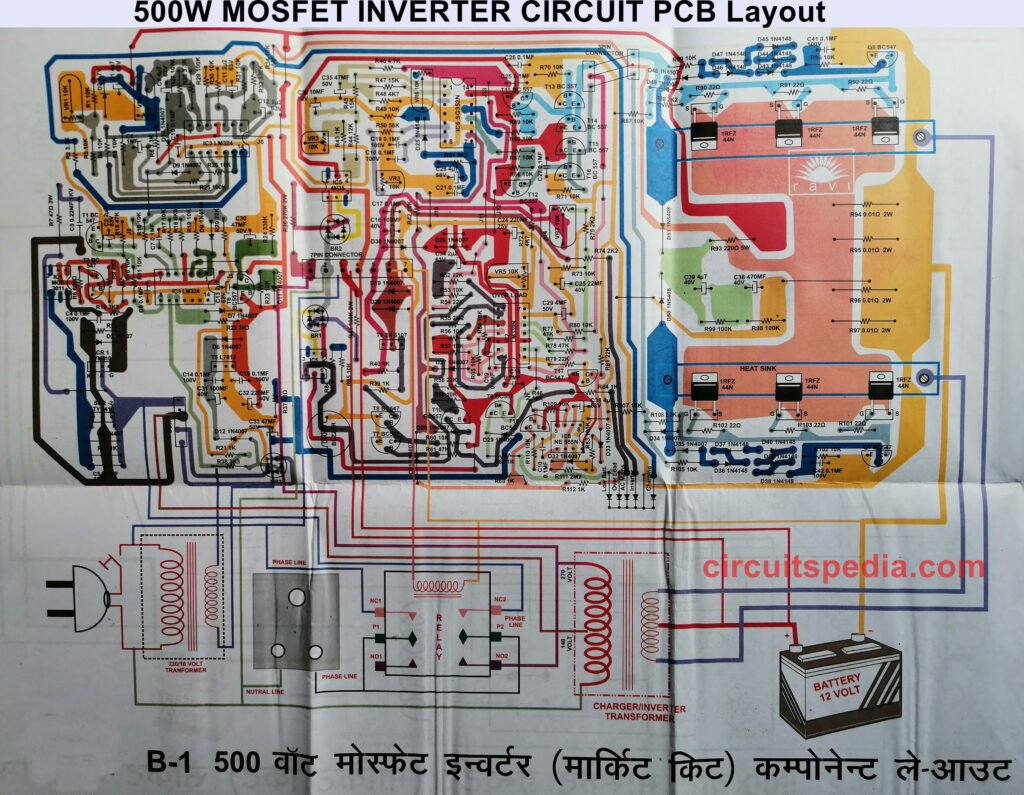Inverter Circuit500W high power inverter circuit diagram

This is the circuit diagram of high power 500W inverter. Below is also component Layout/PCB layout of this schematic diagram. circuit diagram PCB Layout/Component Layout   Related Post Microtek digital inverter circuit Switch ON Delay Timer circuit Stereo Audio Amplifier Circuit Using CD6283 Top10 electronic circuit diagram for beginners Luminous digital inverter circuit diagram300W inverter circuit diagram

This is the circuit diagram of a 300W simple inverter. This inverter circuit uses two ic NE555 and SN74LS112 and 10 2N3055 Transistor with some other components.   Also Read Stabilizer Circuit Diagram Su-Kam digital inverter circuit diagram Top 5 Circuit diagram For Beginners Arduino Remote control AC Dimmer Light Activated Switch using TransistorInverter with charger circuit diagram (4 in 1)

12v DC to 220v AC Inverter with battery charger circuit diagramSu-Kam digital inverter circuit diagram

Sukam (Su-Kam) digital inverter circuit diagram. 560VA/12V sukam digital inverter circuit digram.High power inverter with charger circuit diagram

This is the complete circuit diagram of a 1250VA/24V MOSFET inverter with battery charger. The PCB layout of this circuit diagram is below.Luminous digital inverter circuit diagram

Luminous digital inverter circuit diagram 750VA. Specification of this popular inverter AC mains LOW/HIGH voltage protection Overload protection Low battery protection capacity to soft start of heavy loads battery charging in four stepsMicrotek digital inverter circuit

Microtek digital inverter circuit diagram This is the circuit diagram of the 550VA Microtek digital inverter. This is the complete circuit diagram and for the PCB layout of this circuit click on the following link. Click here to Download PCB layout In Full HD2000W inverter circuit

2000W Inverter circuit diagram This is the circuit diagram of 2000w high power inverter circuit. This is based on the mosfet3205 . use 24v DC supply for operation and connect 24v 5A or more than 5A transformer. Please careful with this circuit because high A voltage. Click on image for best resolution   Click to …CFL Driver Inverter Circuit

12v CFL Inverter circuit for Emergency Light This is a small power inverter circuit that produces 230v ac at the output through the transformer. The circuit is designed by T.Kalpana. This can be used as the emergency CFL lighting, CFL needs AC input for operating and this circuit provides the AC to CFL. This circuit …